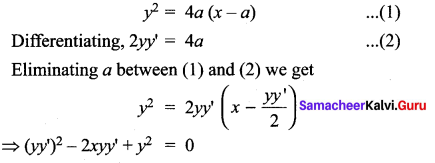# Samacheer Kalvi 12th Maths Solutions Chapter 10 Ordinary Differential Equations Ex 10.3

## Tamilnadu Samacheer Kalvi 12th Maths Solutions Chapter 10 Ordinary Differential Equations Ex 10.3

Question 1.
Find the differential equation of the family of
(i) all non-vertical lines in a plane
Solution:
Equation of family of all non-vertical lines is
y = mx + c (m ≠ 0)
Differentiate with respect to ‘x’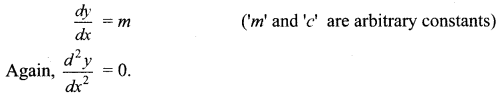(ii) all non-horizontal lines in a plane.
Solution:
Equation of family of all non-horizontal lines is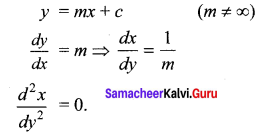Question 2.
Form the differential equation of all straight lines touching the circle x2 + y2 = r2
Solution:
Equation of circle x2 + y2 = r2 of the line y = mx + c is to be a tangent to the circle, then the equation of the tangent is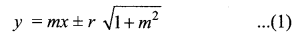Differentiating with respect to V dy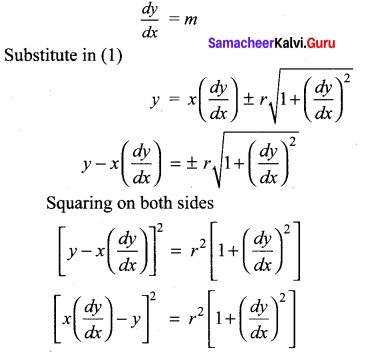Question 3.
Find the differential equation of the family of circles passing through the origin and having their centres on the x -axis.
Solution:
All circles passing through the origin and having their centre on the x -axis say at (a, 0) will have radius ‘a’ units.
∴ Equation of circle is (x – a2) + y2 = a2 ….(1) [ ∵ ‘a’ arbitrary constant]
Differentiate with respect to ‘x’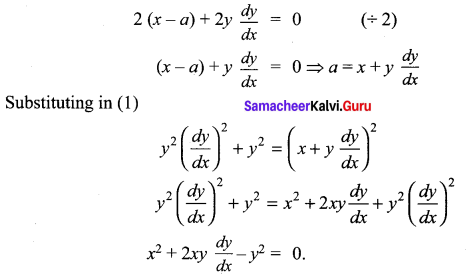Question 4.
Find the differential equation of the family of all the parabolas with latus rectum 4a and whose axes are parallel to the x -axis.
Solution:
Equation of all parabolas whose axis is parallel to X – axis is
(y – k)2 = 4a (x – h)
Where (h, k) is the vertex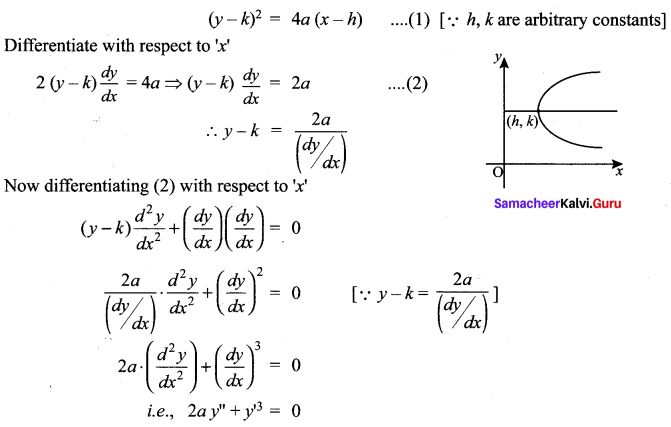Question 5.
Find the differential equation of the family of parabolas with vertex at (0, -1) and having axis along the y – axis.
Solution:
Given, vertex (0, -1) and axis along y-axis
Equation of Parabola, (x + 1)2 = – 4ay …… (1) [∵ a is the perameter]
Differentiate with respect to ‘x’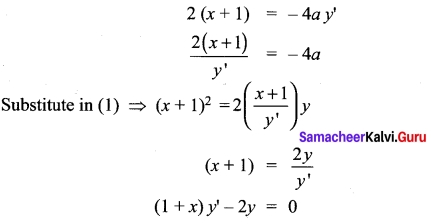Question 6.
Find the differential equations of the family of all the ellipses having foci on the y – axis and centre at the origin.
Solution:
Equations of the family of all the Ellipses having foci on the y – axis and centre at the origin is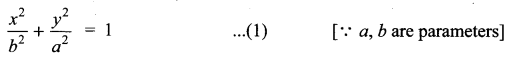Differentiate with respect to ’x’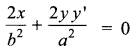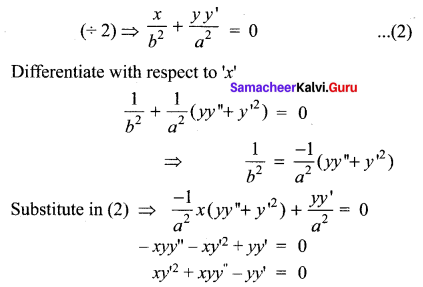Question 7.
Find the differential equation corresponding to the family of curves represented by the equation y = Ae8x + Be-8x, where A and B are arbitrary constants.
Solution: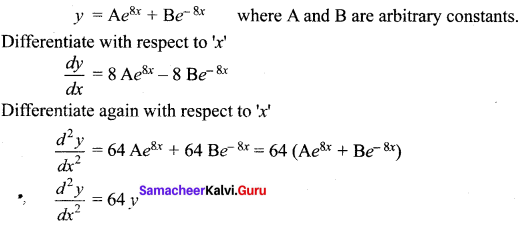Question 8.
Find the differential equation of the curve represented by xy = aex + be-x + x2.
Solution:
xy = aex + be-x + x2
xy – x2 = aex + be-x …… (1) [∵ a’, b’ are arbitrary constants]
Differentiate with respect to ‘x’
xy’ +y – 2x = aex – be-x
Again, Differentiate with respect to ‘x’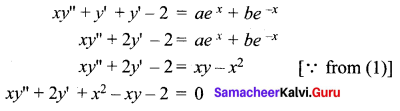### Samacheer Kalvi 12th Maths Solutions Chapter 10 Ordinary Differential Equations Ex 10.3 Additional Problems

Question 1.
Find the differential equation of the family of straight lines y = mx + $$\frac{a}{m}$$ when
(i) m is the parameter,
(ii) a is the parameter,
(iii) a, m both are parameters.
Solution: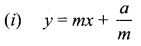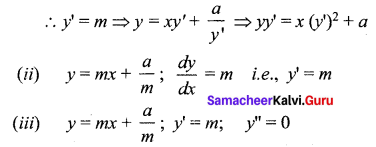Question 2.
Find the differential equation that will represent family of all circles having centres on the x-axis and the radius is unity.
Solution:
Equation of a circle with centre on x-axis and radius 1 unit is
(x – a)2 + y2 = 1 ….. (1)
Differentiating with respect to x,
2 (x – a) + 2yy’ – 0
⇒ 2 (x – a) = – 2yy’
(or) x – a = -yy’ ……(2)
Substituting (2) in (1), we get,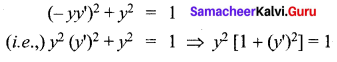Question 3.
From the differential equation from the following equations.
(i) y = e2x (A + Bx)
Solution:
ye-2x = A + Bx ……. (1)
Since the above equation contains two arbitrary constants, differentiating twice,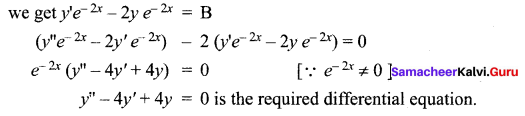(ii) y = ex(A cos 3x + B sin 3x)
Solution: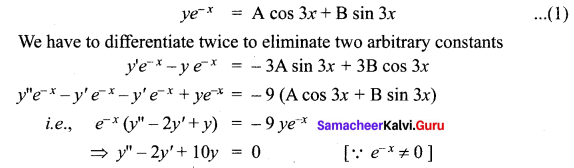(iii) Ax2 + By2 = 1
Solution: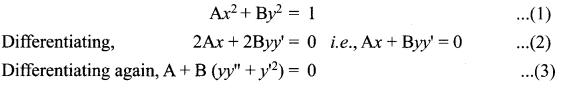Eliminating A and B between (1), (2) and (3) we get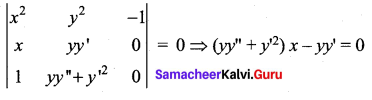(iv) y2 = 4a(x – a)
Solution: